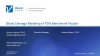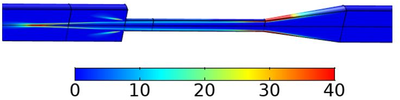# Technical Papers and Presentations

## Blood Damage Modeling of FDA Benchmark Nozzle

A. Kermani1, A. Vanegas1, A. Spann1
1Veryst Engineering LLC., Needham, MA, USA
Published in 2020

Hemolysis caused by flow-induced mechanical damage to red blood cells is a concern in devices that involve transporting blood. Mathematical models have been proposed to estimate red blood cell damage in blood flow. We used hemolysis mathematical models to evaluate the effect of modifications to the device geometry on blood damage. We used Lagrangian and Eulerian approaches to obtain blood damage estimates based on the following equations in Ref. . (∂Db)/∂t+(V.∇) Db=τ^(α/β) ∂H/∂t+(V.∇)H=〖cβD_b^(β-1) τ〗^(α/β)

Here H is the ratio of released hemoglobin to total hemoglobin within the red blood cells, t is time, V is velocity, τ is shear stress, C, α, and β are constant parameters based on experimental data, D_b is mechanical dose. Mechanical dose accounts for accumulated blood damage based on the shear stress that is experienced by the red blood cells .

For Eulerian implementation of the above equations, we used the Transport of Diluted Species physics interface in COMSOL Multiphysics®. The right-hand sides of the equations are defined as reaction terms. For Lagrangian implementation, we used the auxiliary dependent variable feature of Particle Tracing for Fluid Flow physics interface in COMSOL® to solve the above equations along path lines of red blood cells. We defined Db and H as auxiliary dependent variables with the following governing equations: dDb=τ^(α/β) dt dH=cβDb^(β-1) dDb

The United States Food and Drug Administration (FDA) initiated benchmark models of typical device flow geometries, including a nozzle . Multiple laboratories participated and provided experimental velocities, pressure, and hemolysis data to support CFD simulations. We used the FDA nozzle shown in Figure 1 as our benchmark. Our preliminary results show that the simple power law stress relation used in this hemolysis model in both its Lagrangian and Eulerian implementations cannot accurately quantify damage at flow rates of 6L/min as used in the FDA experiments. However, the models can predict which geometries are more prone to blood damage than others. Therefore, these models can be used to compare hemolysis in different device geometries and assess the effect of slight geometrical modifications.